# Balanced circulants

Given interer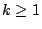and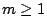, let us introduce a graph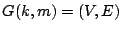with circular symmetry as follows: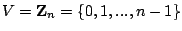, where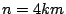, and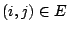iff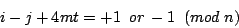for some integer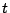. E.g. if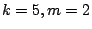then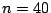andiff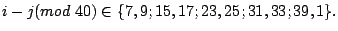It is not difficult to check that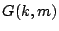is balanced, i.e. it has no odd cycles, nor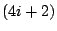-holes. In fact, it may only contain holes of length 4 and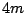. Moreover, every-cycle has at least two chords. Hence,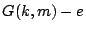is still balanced for any edge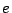, in agreement with Conjecture 2 from the section "Balanced Graphs". Conjecture 1 of the same section also holds for. Indeed, if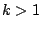then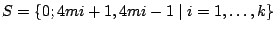is a star cutset: 0 is an isolated vertex in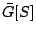, while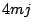is an isolated vertex in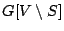for every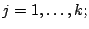and if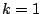thenis-cycle, that is a basic graph

CONJECTURE. Every non-empty balanced circulant is isomorphic to a.